Birch and Swinnerton-Dyer Conjecture Clay Institute Millenium Problem Solution | OMICS International
Journal of Physical Mathematics
All submissions of the EM system will be redirected to Online Manuscript Submission System. Authors are requested to submit articles directly to Online Manuscript Submission System of respective journal.

# Birch and Swinnerton-Dyer Conjecture Clay Institute Millenium Problem Solution

Cusack P*

Corresponding Author:
Cusack P
Independent Researcher
Tel:(506)214-3313
E-mail: [email protected]

Received date: February 08, 2016; Accepted date: April 29, 2016; Published date: May 05, 2016

Citation: Cusack P (2016) Birch and Swinnerton-Dyer Conjecture Clay Institute Millenium Problem Solution. J Phys Math 7:172. doi:10.4172/2090-0902.1000172

Copyright: © 2016 Cusack P. This is an open-access article distributed under the terms of the Creative Commons Attribution License, which permits unrestricted use, distribution, and reproduction in any medium, provided the original author and source are credited.

Visit for more related articles at Journal of Physical Mathematics

#### Abstract

This paper presents the solution to the Birch Swimmerton problem. It entails the use of critical damping of a Mass-Spring-Dash Pod system which, when modelled mathematically, provide the equation that allows the solution of the zeta problem to be solved.

#### Keywords

Zeta functions; Dash pod systems; Mean functions

#### Introduction

But in special cases one can hope to say something. When the solutions are the points of an abelian variety, the Birch and Swinnerton- Dyer conjecture asserts that the size of the group of rational points is related to the behavior of an associated zeta function ζ(s) near the point s=1. In particular this amazing conjecture asserts that if ζ(1) is equal to 0, then there are an infinite number of rational points (solutions), and conversely, if ζ(1) is not equal to 0, then there is only a finite number of such points .

#### Explanation

Equation of motion

From Verruijt, we know the equation of motion for a mass –springdashpod system is:

m*d2u/dt2+c*du/dt+ku=0

So, taking the resonant frequency into account, the equation from Verruijt becomes:

d2u/dt2+2zw0*du/dt+w02u=0

Where w0=resonant frequency and z is a measure of the system damping .

At critical damping, the characteristic equation is the golden mean function:

x−=1/[x−1]

Or,

x2−x−1=0

The roots to this equation are, of course, -0.618 and 1.618.

Value for i-the imaginary number Now, before examining zeta z in equation form, we calculate a real value for the imaginary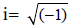[1−i]=1/[(1−i)−1]

1−i=1/−i

−i=1/[1−i]

i=1/[i−1]

x=1/[x−1]

x=-0.618, 1.618

So,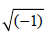= -0.618, 1.618

Damping ratio zeta z

Now, zeta=z=damping ratio=w/w0:

du0 / dw = 0 : w / w0 =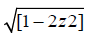Algebraically:

du0/0=dw

w = w0*Taking the derivative:

du0/0=dw=w′=[w0*(1−2z2)1/2]′

w0/2*(1−2z2)1.5]/1.5

In the Birch conjecture, there are two possibilities to consider . They are:

z(1)=0 and z(1)(not=)0

In the first case:

0=w0/3[(1−2(1)2]1.5

0=w0/3(11.5)

W0=0

Z(1)=0,w0=0

Critical damping

In the second case, we have critical damping. z(1) (not=)m 0

Say z(1)=1

1=w0/3[(1−2(12)]1.5 w0=3

Or w0=C1 w0 is a real number. In case 1 again:

Z(1)=0,w0=0

du / dw = 0w / w0 =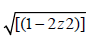w / 0 = [(1− 2(z)2)] w=0

w/w0=0/0 Dividing by zero has infinite solution. Now, finally, in the critical damping case: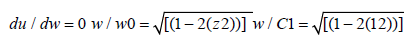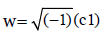We know =-0.618, 1.618. So, w=-0.618 0r 1.618 w/w0=0.618 C1/C1=0.618. Therefore there is a real solution to z at critical damping [4,5].

#### Conclusion

Simple Mechanics combined with knowledge of the zeta function and the value of the imaginary number provides the ingredients to solve the Birch and Swinnerton-Dyer Conjecture.

#### References

Select your language of interest to view the total content in your interested language

### Article Usage

• Total views: 8587
• [From(publication date):
June-2016 - Nov 18, 2019]
• Breakdown by view type
• HTML page views : 8445Can't read the image? click here to refresh